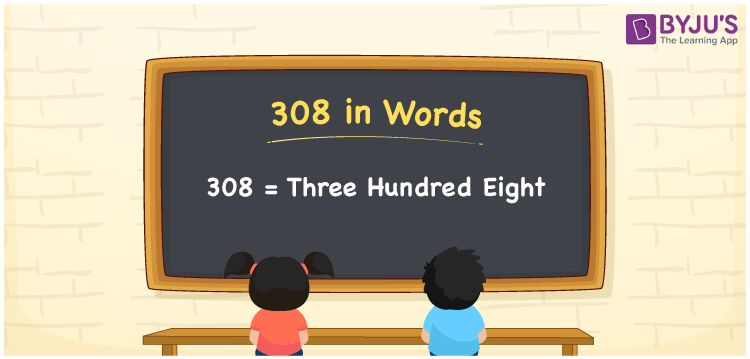# 308 in Words

308 in words can be written as Three Hundred Eight. In this article, you will understand the concept of count or counting which is very important in Mathematics. If you pay Rs. 308 for internet connection per month, then you can say that “I paid Three Hundred Eight Rupees for internet connection per month”. Learn the place value of 308 using the chart given for the reference of students. So, the number 308 can be read as “Three Hundred Eight” in words.

 308 in words Three Hundred Eight Three Hundred Eight in Numbers 308

## 308 in English Words## How to Write 308 in Words?

Get to know the correct method of writing numbers in words. Use the chart below and convert numbers in the English alphabet.

 Hundreds Tens Ones 3 0 8

308 in expanded form can be written as:

3 × Hundred + 0 × Ten + 8 × One

= 3 x 100 + 0 x 10 + 8 x 1

= 300 + 8

= 308

= Three Hundred Eight

Hence, 308 in words is written as Three Hundred Eight.

308 is a natural number that precedes 309 and succeeds 307.

308 in words – Three Hundred Eight

Is 308 an odd number? – No

Is 308 an even number? – Yes

Is 308 a perfect square number? – No

Is 308 a perfect cube number? – No

Is 308 a prime number? – No

Is 308 a composite number? – Yes

## Frequently Asked Questions on 308 in Words

Q1

### How to write 308 in words?

308 can be written in words as “Three Hundred Eight”.
Q2

### Write Three Hundred Eight in numbers.

Three Hundred Eight in numbers can be written as 308.
Q3

### Is 308 an even number?

308 is an even number as it is completely divisible by 2.